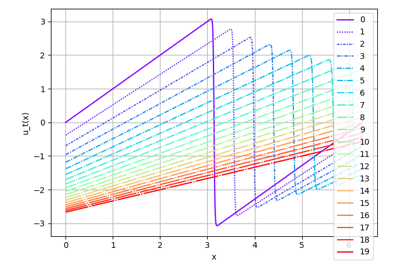gemseo / post / dataset

# curves module¶

Draw curves from a Dataset.

A Curves plot represents samples of a functional variable $$y(x)$$ discretized over a 1D mesh. Both evaluations of $$y$$ and mesh are stored in a Dataset, $$y$$ as a parameter and the mesh as a misc.

class gemseo.post.dataset.curves.Curves(dataset, mesh, variable, samples=None)[source]

Bases: DatasetPlot

Plot curves y_i over the mesh x.

Parameters:
• dataset (Dataset) – The dataset containing the data to plot.

• mesh (str) – The name of the dataset misc corresponding to the mesh.

• variable (str) – The name of the variable for the x-axis.

• samples (Sequence[int] | None) – The indices of the samples to plot. If None, plot all the samples.

Raises:

ValueError – If the dataset is empty.

execute(save=True, show=False, file_path='', directory_path=None, file_name=None, file_format=None, properties=None, fig=None, axes=None, **plot_options)

Execute the post-processing.

Parameters:
• save (bool) –

If True, save the plot.

By default it is set to True.

• show (bool) –

If True, display the plot.

By default it is set to False.

• file_path (str | Path) –

The path of the file to save the figures. If empty, create a file path from directory_path, file_name and file_format.

By default it is set to “”.

• directory_path (str | Path | None) – The path of the directory to save the figures. If None, use the current working directory.

• file_name (str | None) – The name of the file to save the figures. If None, use a default one generated by the post-processing.

• file_format (str | None) – A file format, e.g. ‘png’, ‘pdf’, ‘svg’, … If None, use a default file extension.

• properties (Mapping[str, DatasetPlotPropertyType] | None) – The general properties of a DatasetPlot.

• fig (None | Figure) – The figure to plot the data. If None, create a new one.

• axes (None | Axes) – The axes to plot the data. If None, create new ones.

• **plot_options – The options of the current class inheriting from DatasetPlot.

Returns:

The figures.

Raises:

AttributeError – When the name of a property is not the name of an attribute.

Return type:

list[Figure]

color: str | list[str]

The color(s) for the series.

If empty, use a default one.

colormap: str

The color map.

dataset: Dataset

The dataset to be plotted.

fig_size: FigSizeType

The figure size.

property fig_size_x: float

The x-component of figure size.

property fig_size_y: float

The y-component of figure size.

font_size: int

The font size.

property labels: Mapping[str, str]

The labels of the variables.

legend_location: str

The location of the legend.

linestyle: str | list[str]

The line style(s) for the series.

If empty, use a default one.

marker: str | list[str]

The marker(s) for the series.

If empty, use a default one.

property output_files: list[str]

The paths to the output files.

title: str

The title of the plot.

xlabel: str

The label for the x-axis.

xmax: float | None

The maximum value on the x-axis.”.

If None, compute it from data.

xmin: float | None

The minimum value on the x-axis.

If None, compute it from data.

xtick_rotation: float

The rotation angle in degrees for the x-ticks.

ylabel: str

The label for the y-axis.

ymax: float | None

The maximum value on the y-axis.

If None, compute it from data.

ymin: float | None

The minimum value on the y-axis.

If None, compute it from data.

zlabel: str

The label for the z-axis.

zmax: float | None

The maximum value on the z-axis.

If None, compute it from data.

zmin: float | None

The minimum value on the z-axis.

If None, compute it from data.

## Examples using Curves¶Burgers dataset

Burgers dataset